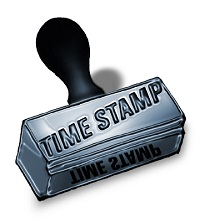# PHP Timestamp

PHP provides several date time functions to perform required operations with temporal data. Now, we are going to see about PHP timestamp functions. The timestamp is the value represented as seconds calculated, since UNIX Epoch, January 1, 1970, and also called as UNIX timestamp.

In PHP, it includes several functions to work with a timestamp. In this article, we are going to see about how the following list of timestamp related functionalities is obtained by using PHP date time functions.

• getting current timestamp
• date/time to timestamp conversion## Getting Current Timestamp in PHP

We can get current timestamp value in three possible ways with the help of PHP core functions described here.

### time()

This is the simple and widely used PHP function to get current timestamp value. It requires no arguments to be sent for returning expected resultant UNIX timestamp. The usage of this simple function is shown in the example PHP program below.

```<?php
\$current_timestamp = time();
echo \$current_timestamp;
?>
```

### strtotime()

This function is mainly used to get timestamp value from the given string representing date value. PHP provides a list of supported strings to be passed as an argument of this function to denote date values. For example, “Tuesday last week”, “+1 week”, “21 November 2008” and etc.

similarly, for getting current timestamp value, we need to provide the string as “now” representing current date and time value. So, the code will be as follows.

```<?php
\$current_timestamp = strtotime("now");
echo \$current_timestamp;
?>
```

While invoking strtotime() by passing improper string data which is not supported by PHP, this function will return false.

### mktime()

This function is also used to get UNIX timestamp but requires set of parameters denoting date components, like an hour, minute, second, month, day, year, in the same order specified here. And also have an optional flag representing daylight saving time state.

And for getting, the current timestamp, we have to use the PHP date() function within this function, with the corresponding parameter for getting the current hour, minute, in required order. For example,

```<?php
\$current_timestamp_by_mktime = mktime(date("H"),date("i"),date("s"),date("m"),date("d"),date("Y"));
echo \$current_timestamp_by_mktime;
?>
```

### microtime()

All the above PHP timestamp functions will return only the 10 digit timestamp value. But, while executing microtime() function, it will return the number of second elapsed since, UNIX Epoch, and also, the number of microseconds elapsed since seconds value returned by this function.

microtime() is same as time() functions, which doesn’t require any parameter, but, there is an optional parameter \$get_as_float. This parameter will accept boolean values for it. If it is TRUE, then microtime() will return float value representing accurate timestamp, otherwise, will return “microseconds seconds” formatted string. And the code is,

```<?php
\$current_timestamp_string = microtime();
echo \$current_timestamp_string;
\$current_timestamp_float = microtime(TRUE);
echo \$current_timestamp_float;
?>
```

### date()

PHP supports several date format characters denoting components of the date value. So, we can use these list of date formatting characters to get date components or to format date if required, using given temporal data.

From the list of those string, U denotes UNIX timestamp value. So, for date() function, we should specify U as an argument to get current timestamp value. For example,

```<?php
\$current_timestamp_fndate = date("U");
echo \$current_timestamp_fndate;
?>
```

## Date/Time to Timestamp Conversion

strtotime() and mktime() functions are also used to convert specified date into the form of a timestamp.

For using strtotime(), we need to pass the date with any one of PHP supported date format, for example, dd/mm/yyyy, mm/dd/yyyy and etc. And, for using mktime(), we need to explode the given date and send the exploded components to this function.

And, we can perform the reverse, that is, converting timestamp value to date, by the use of date() function. For that, we should specify the required date format as the first parameter, and timestamp as the second one. For example,

```<?php
\$date_from_timestamp = date("d-m-Y",\$current_timestamp);
echo "Formatted date from timestamp:" . \$date_from_timestamp;
?>
```

Note:

• These operations can also be performed in the object oriented style of programming, with the PHP functions defined under DateTime class interface.
• On the other hand, certain PHP timestamp functions are in procedural style, which is the alias of class constructors defined for PHP DateTime object model.